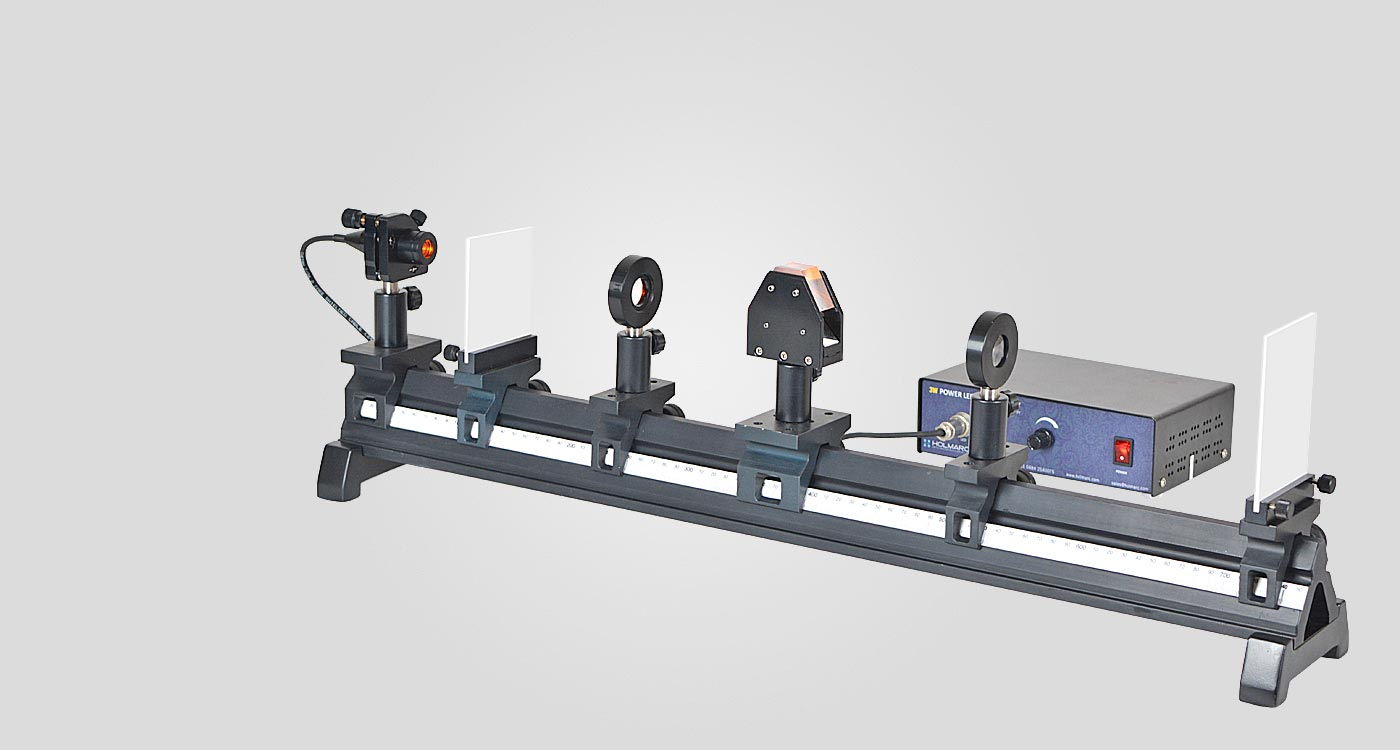Refractometer

Model: HO-ED-O-03

Holmarc's Refractometer (Model No: HO-ED-O-03) is designed for understanding the basics of refractometry. It can visualize the output on a screen. One can do the calibration and can obtain the refractive index of various types of liquids.

A refractometer is a laboratory device used for the measurement of 'index of refraction'. This refractometer utilizes the concepts of critical angle and total internal reflection to measure the refractive index of samples.

In this experiment, the sample is placed on the dove prism. Dove prisms rotate an image without deviating beam in such a way that the image rotates at twice the angular rate of the prism. LED light of wavelength 589nm is collimated and directed to the interface between the prism and the solution. The light rays meet the interface at different angles. Some of these angles are larger than the critical angle of the interface so that light is totally reflected. For angles of incidence smaller than the critical angle, light is partially transmitted (i.e. lost). Thus a shadow (less luminescence) is created on the screen. The position of the shadow indicates the magnitude of the critical angle. Hence the refractive index can be obtained.

Measurement is performed on a sugar solution with known sugar concentration. Then the refractive index of a solution with an unknown concentration is measured. Through a comparison with the initial measurement, the unknown concentration can be deduced. In liquids and solids, the speed of light varies significantly with wavelength and the index of refraction . For the most accurate measurements it is necessary to use monochromatic light. Bright LED light is used here. Fine adjustments in grazing or incident angle can be done using kinematic tuning of the LED mount.

The lenses and dove prism are fixed on rail carriers which are held on graduated solid aluminum rail. All the mounts are made of anodized aluminum and can be mounted easily on the optical rail.

Experiment Examples

To find the refractive index of an unknown solution.

From Snell’s law,

sin i / sin r = n2 / n1

Light incident on the travels from the first medium (prism) to the second medium (sample). So n1 is the refractive index of the prism. The material of the prism is NSF - 1 having a refractive index of 1.717. So n1 = 1.717.

The second medium is the sample material and its index n2. Knowing the refractive index of the sample we can find out the critical angle for the sample-prism interface.

i.e. sin c = ( n2 / n1 ) sin 90

sin c = n2 / 1.717

Then find the refractive index using,

n2 = sin c x 1.717

Note: Use samples of refractive index 1.40 < n2 < 1.60.Fig. Schematic diagram

Features

Refractive index measurement is easy and efficient

Compact and precision design

High performance LED light source of optical wavelength 589nm

Precise optics minimize aberrations

Can observe clear and sharp shadow limits

Mounts and rail with black anodized finish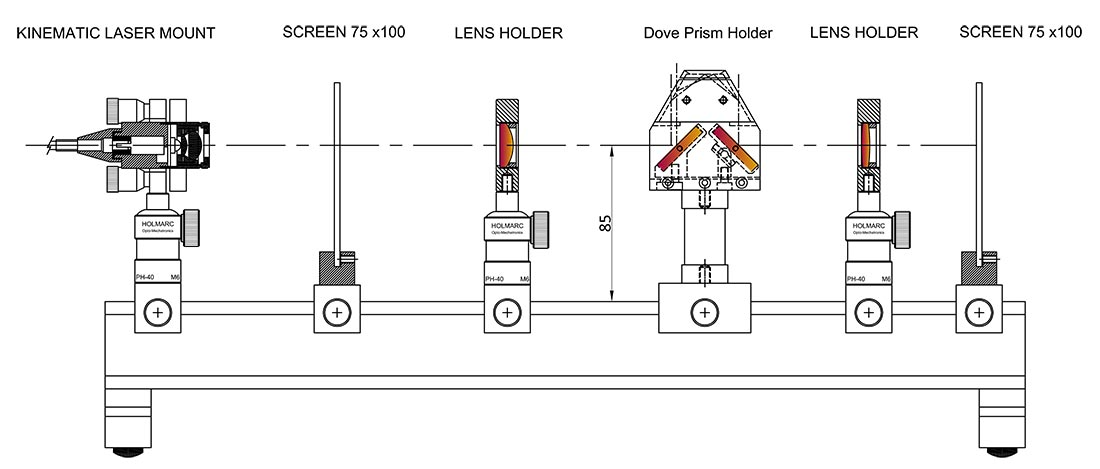Related Topics

Index of refraction

Reflection by dove prism

Total Internal reflection

Dispersion

Scope of Supply

Optical Rail

Model No: ED-O-03-OR
Length
:
750 mm
Material
:
Anodized Aluminium Alloy
Quantity
:
1 no.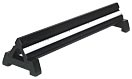Kinematic Laser Mount

Model No: ED-O-03-KLM
Material
:
Black anodized Aluminum alloy
:
:
+/-4 degrees
Quantity
:
1 no.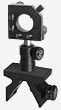Dove Prism with Mount

Model No: ED-O-03-DPM
Material
:
F2
Length
:
50 mm
Quantity
:
1 no.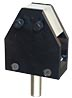Plano Convex Lens with Mount

Model No: ED-O-03-PCLM
Material
:
N - BK7
Diameter
:
20 mm & 25 mm
Focal Length
:
40 mm & 100 mm
Quantity
:
1no. each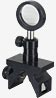White Screen with Mount

Model No: ED-O-03-WSM
Material
:
Acrylic
Quantity
:
1 no.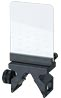LED with Variable Power supply (White)

Model No: ED-O-03-LEDP
Output Power
:
0 - 3 Watt Variable
Wavelength
:
589 nm
Quantity
:
1 no.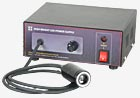Accessories

Allen Key
User Manual

|